### Home > PC3 > Chapter 8 > Lesson 8.1.2 > Problem8-38

8-38.
1. Take the reciprocal of each of the following fractions and then simplify. Homework Help ✎

1.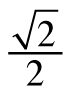2.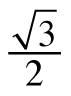3. 0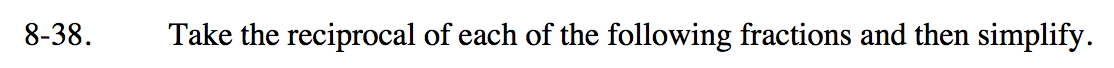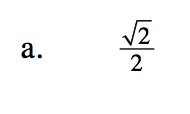$\frac{2}{\sqrt{2}}\cdot \frac{\sqrt{2}}{\sqrt{2}}$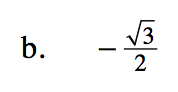Follow similar steps as shown in part (a).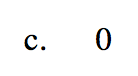What is the value of 1/0?